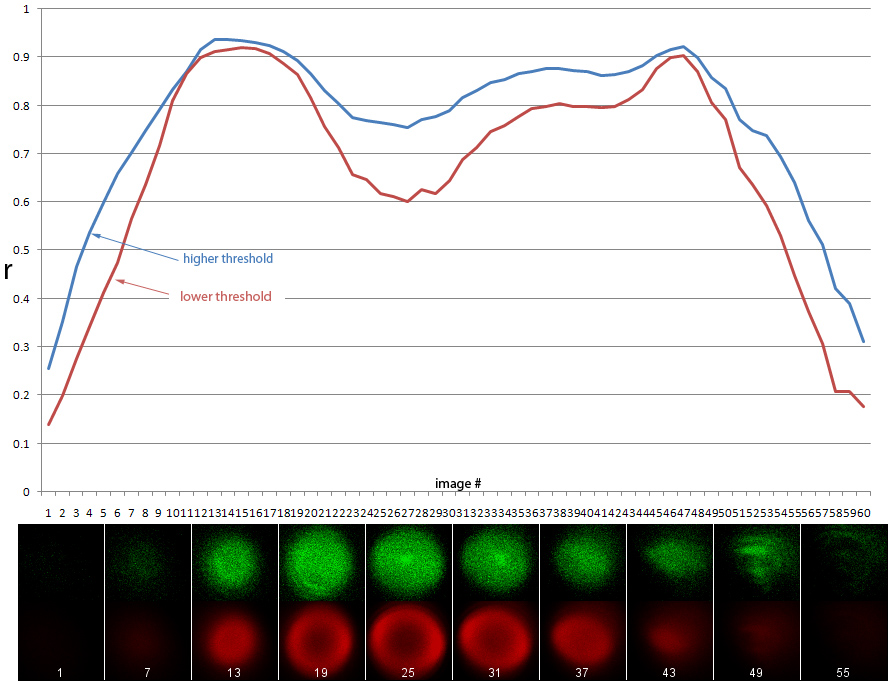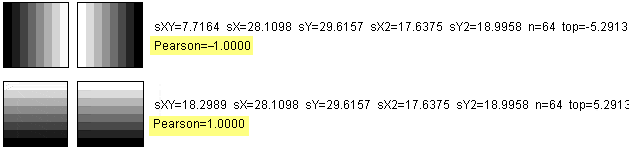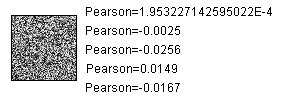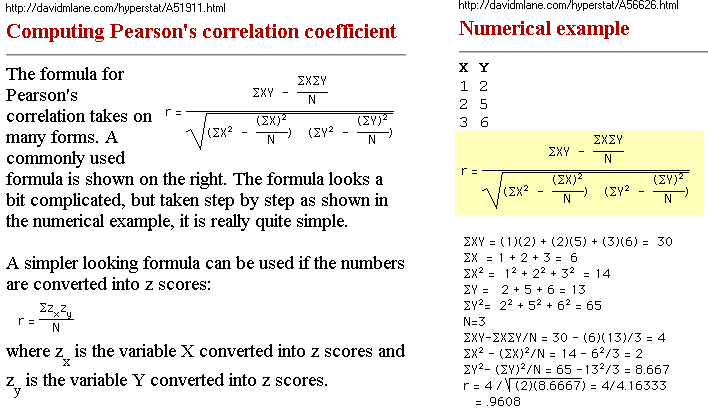Pearson's correlation (r) on a stack of images

ImageJ macro Pearson_on_stack_v400.txt

Example:Implements:

Images that are identical have a coefficient of 1.
Images that are inverted from each other have a coefficient of -1.And images generated randomly approach zero.Although these examples are with synthetic images, I also tested this with fluorescence microscopy images from a cooled CCD camera that were
1. converted to 32 bits (floating point) and
2. divided by 4096 to avoid explosion to infinity using the following formula:<-Go Back